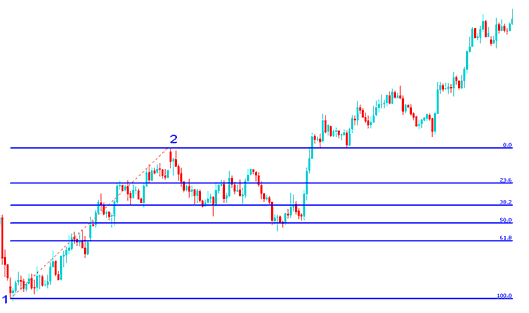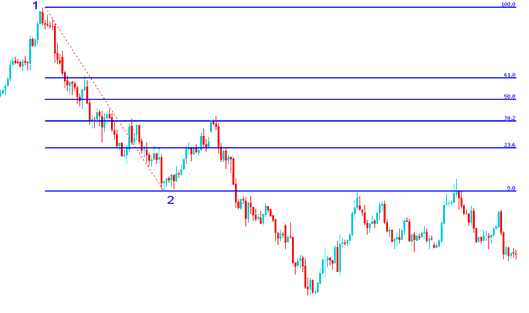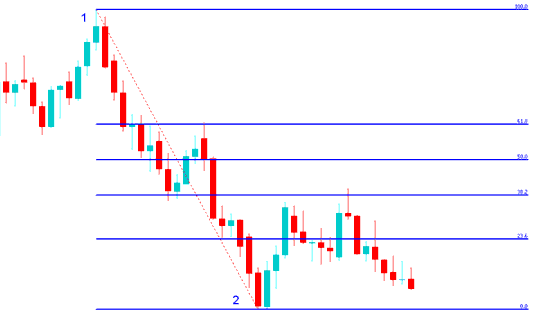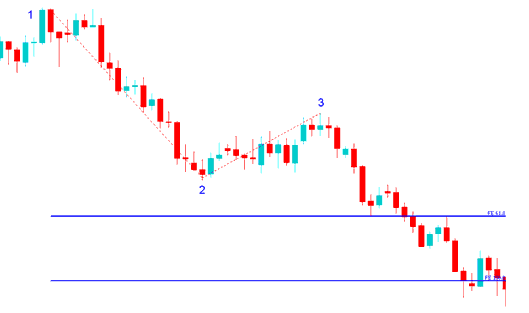# Fibonacci Indices Exercises

These exercises will give more examples on retracement and expansion. The first exercise is to figure out the direction of the Indices trend and then determine if the Fibonacci tool used is retracement or expansion and then figure how it is drawn then practice drawing them on a indices chart.

1. Figure out the direction of the Indices trend

2. Determine if the Fibonacci is retracement or expansion

3. Figure how it is drawn

4. Practice drawing retracement or expansion on a stock indexes trading chart.

After you have downloaded MT4, Go to the menu "insert" at the top left corner, on the drop down menu, click Fibonacci and select either the option of retracement or expansion. Then draw it as explained in the previous lessons of Expansion and retracement. Then do the following exercises:

## Exercises 1:Exercises 2:Exercises 3:Exercises 4:Exercises 5:Hint: point 1 to point 2 follows the indices trend direction

Hint: Retracement has points 1 and 2

Hint: Expansion has point 1, 2 and 3